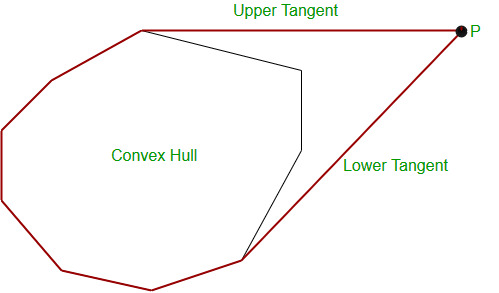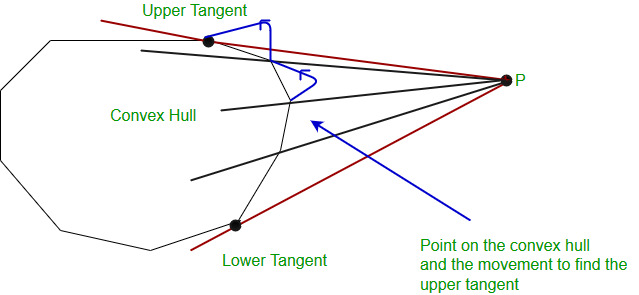# Dynamic Convex hull | Adding Points to an Existing Convex Hull

Given a convex hull, we need to add a given number of points to the convex hull and print the convex hull after every point addition. The points should be in anti-clockwise order after addition of every point.
Examples:

```Input :
Convex Hull : (0, 0), (3, -1), (4, 5), (-1, 4)
Point to add : (100, 100)

Output :
New convex hull : (-1, 4) (0, 0) (3, -1) (100, 100)
```

## Recommended: Please try your approach on {IDE} first, before moving on to the solution.

We first check whether the point is inside the given convex hull or not. If it is, then nothing has to be done we directly return the given convex hull. If the point is outside the convex hull, we find the lower and upper tangents, and then merge the point with the given convex hull to find the new convex hull, as shown in the figure.The red outline shows the new convex hull after merging the point and the given convex hull.

To find the upper tangent, we first choose a point on the hull that is nearest to the given point. Then while the line joining the point on the convex hull and the given point crosses the convex hull, we move anti-clockwise till we get the tangent line.The figure shows the moving of the point on the convex hull for finding the upper tangent.

Note: It is assumed here that the input of the initial convex hull is in the anti-clockwise order, otherwise we have to first sort them in anti-clockwise order then apply the following code.

## Recommended: Please try your approach on {IDE} first, before moving on to the solution.

Code:

 `// C++ program to add given a point p to a given ` `// convext hull. The program assumes that the ` `// point of given convext hull are in anti-clockwise ` `// order. ` `#include ` `using` `namespace` `std; ` ` `  `// checks whether the point crosses the convex hull ` `// or not ` `int` `orientation(pair<``int``, ``int``> a, pair<``int``, ``int``> b, ` `                ``pair<``int``, ``int``> c) ` `{ ` `    ``int` `res = (b.second-a.second)*(c.first-b.first) - ` `              ``(c.second-b.second)*(b.first-a.first); ` ` `  `    ``if` `(res == 0) ` `        ``return` `0; ` `    ``if` `(res > 0) ` `        ``return` `1; ` `    ``return` `-1; ` `} ` ` `  `// Returns the square of distance between two input points ` `int` `sqDist(pair<``int``, ``int``> p1, pair<``int``, ``int``> p2) ` `{ ` `    ``return` `(p1.first-p2.first)*(p1.first-p2.first) + ` `           ``(p1.second-p2.second)*(p1.second-p2.second); ` `} ` ` `  `// Checks whether the point is inside the convex hull or not ` `bool` `inside(vector> a, pair<``int``, ``int``> p) ` `{ ` `    ``// Initialize the centroid of the convex hull ` `    ``pair<``int``, ``int``> mid = {0, 0}; ` ` `  `    ``int` `n = a.size(); ` ` `  `    ``// Multiplying with n to avoid floating point ` `    ``// arithmetic. ` `    ``p.first *= n; ` `    ``p.second *= n; ` `    ``for` `(``int` `i=0; i> &a, pair<``int``, ``int``> p) ` `{ ` `    ``// If point is inside p ` `    ``if` `(inside(a, p)) ` `        ``return``; ` ` `  `    ``// point having minimum distance from the point p ` `    ``int` `ind = 0; ` `    ``int` `n = a.size(); ` `    ``for` `(``int` `i=1; i=0) ` `        ``up = (up + 1) % n; ` ` `  `    ``// Find the lower tangent ` `    ``int` `low = ind; ` `    ``while` `(orientation(p, a[low], a[(n+low-1)%n])<=0) ` `        ``low = (n+low - 1) % n; ` ` `  `    ``// Initialize result ` `    ``vector>ret; ` ` `  `    ``// making the final hull by traversing points ` `    ``// from up to low of given convex hull. ` `    ``int` `curr = up; ` `    ``ret.push_back(a[curr]); ` `    ``while` `(curr != low) ` `    ``{ ` `        ``curr = (curr+1)%n; ` `        ``ret.push_back(a[curr]); ` `    ``} ` ` `  `    ``// Modify the original vector ` `    ``ret.push_back(p); ` `    ``a.clear(); ` `    ``for` `(``int` `i=0; i > a; ` `    ``a.push_back({0, 0}); ` `    ``a.push_back({3, -1}); ` `    ``a.push_back({4, 5}); ` `    ``a.push_back({-1, 4}); ` `    ``int` `n = a.size(); ` ` `  `    ``pair<``int``, ``int``> p = {100, 100}; ` `    ``addPoint(a, p); ` ` `  `    ``// Print the modified Convex Hull ` `    ``for` `(``auto` `e : a) ` `        ``cout << ``"("` `<< e.first << ``", "` `              ``<< e.second << ``") "``; ` ` `  `    ``return` `0; ` `} `

Output:

```(-1, 4) (0, 0) (3, -1) (100, 100)
```

Time Complexity:
The time complexity of the above algorithm is O(n*q), where q is the number of points to be added.

This article is contributed by Amritya Vagmi and would like to contribute, you can also write an article using contribute.geeksforgeeks.org or mail your article to contribute@geeksforgeeks.org. See your article appearing on the GeeksforGeeks main page and help other Geeks.

Attention reader! Don’t stop learning now. Get hold of all the important DSA concepts with the DSA Self Paced Course at a student-friendly price and become industry ready.

My Personal Notes arrow_drop_up
Article Tags :
Practice Tags :

Be the First to upvote.

Please write to us at contribute@geeksforgeeks.org to report any issue with the above content.